# KnightCTF Reverse Engineering Writeups

### The Flag VaultBasic Info:

• File command• It’s an ELF 64bit executable.
• Strings command• There are some interesting strings and library calls ( strcmp ). So run it and see how it works and it ask us for the password, we give the password string and the output is wrong.• For further analysis, load the binary in gdb and disassemble the main function• Look at the main + 242 line instruction, it compare two strings. Let’s set breakpoint at main+242 and examine the values.• Now run the program with some random string, here i used password as input.• After hitting the strcmp instruction ,examine the two values. First one is the password and the second one is our input. Now run the binary with finded password.• `The Flag is KCTF{welc0me_t0_reverse_3ngineering}`

### The EncoderBasic Info:

• File command• It’s an ELF 64bit executable and not stripped binary.
• Strings command:• Nothing interesting. Now run the binary and see how it works. It print welcome message and ask for plain text to encrypt ( the input length must within in 40 character )Enter hello guys as input, it gives some numbers 🙄. They are given the encrypted flag, now we need to decrypt it.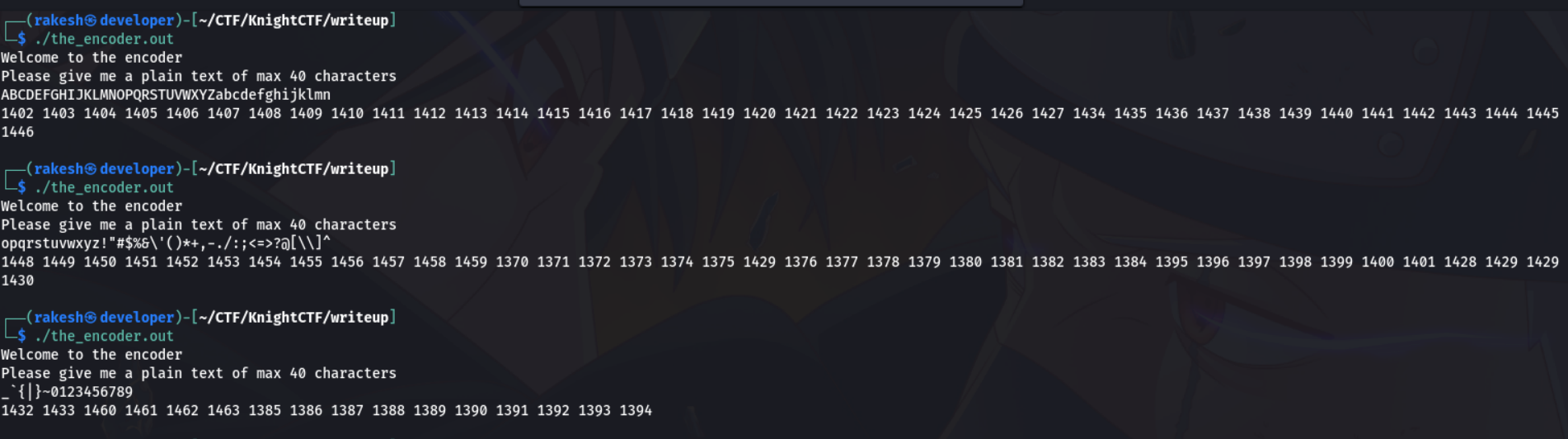Now enter the all printable character as input, it gives the encrypted value for corresponding input. Let’s make a script which find the correct value of flag by its encrypt value.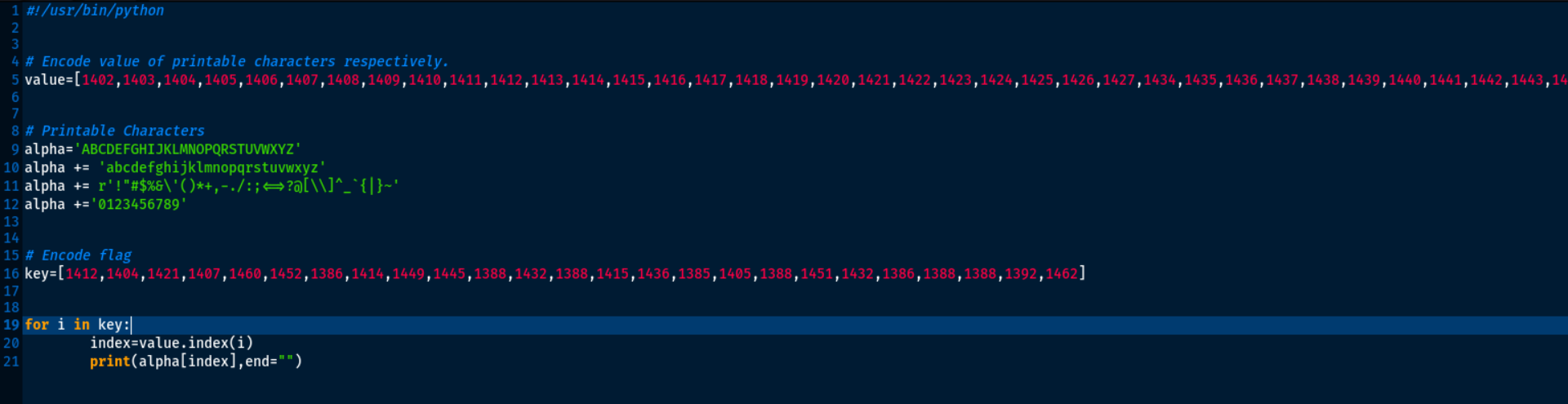For example the first encrypted value is 1412, the script find the index of 1412 in value variable and print the finded index value of alpha. Now run the script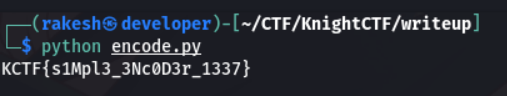The Flag is `KCTF{s1Mpl3_3Nc0D3r_1337}`.

### Baby shark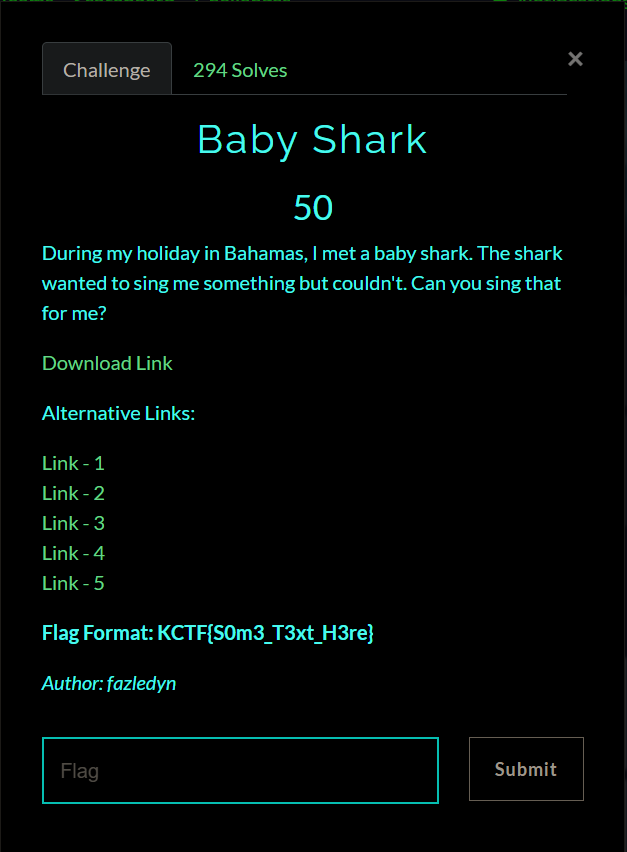##### Basic Info
###### File command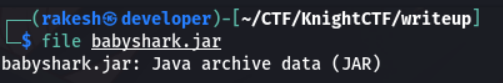It is java archieve data (JAR). Now unzip the jar file and analysis the files.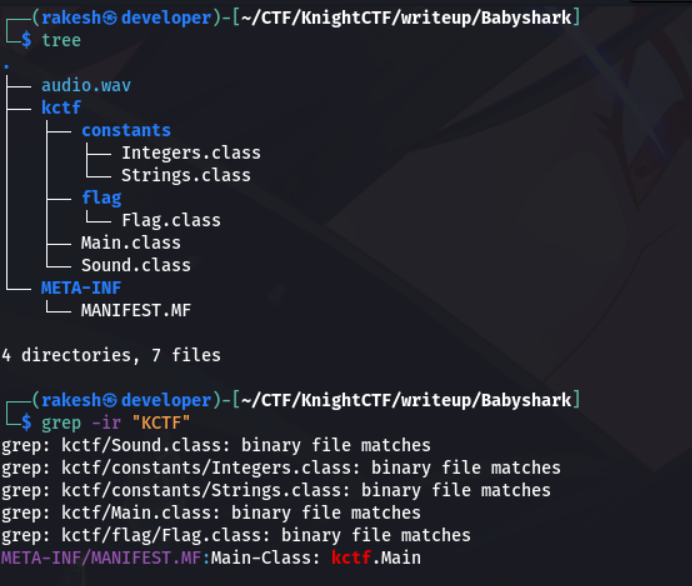There is one audio.wav file, but there is nothing interesting. The flag.class contain nothing. So grep the all files , but there is no flag. Cat the string.class, it contain some base64 encode value. So decode it.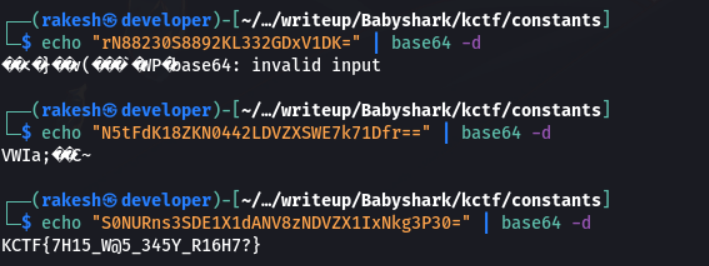Yeah!!! There is flag `KCTF{[email protected]_345Y_R16H7?}`

### Knight Vault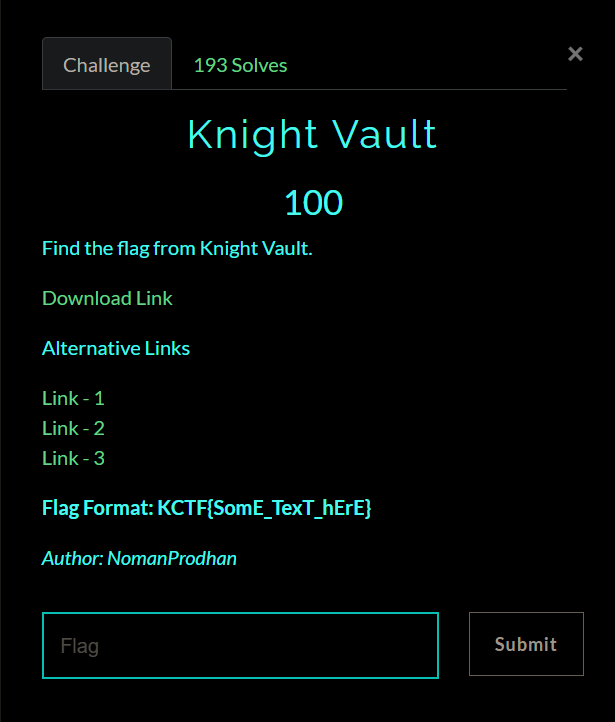##### Basic Info
###### File command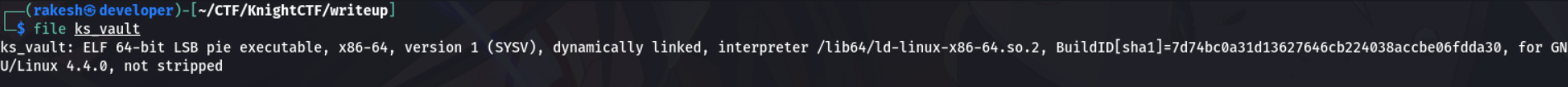It’s an ELF 64bit executable and not stripped binary.

###### Strings command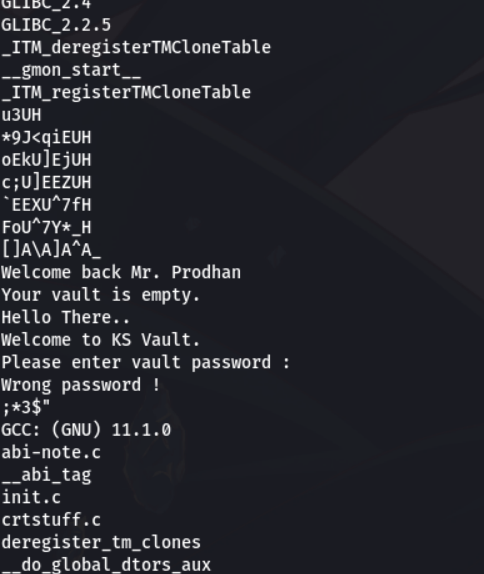Nothing interesting. Now run the binary and see how it works. It print hello message and ask for password.Enter 12345 as input, it gives wrong password. Load the binary in ghidra to analysis. Look at the main function, it take a input from user and modified the input by subtract 10 in given input.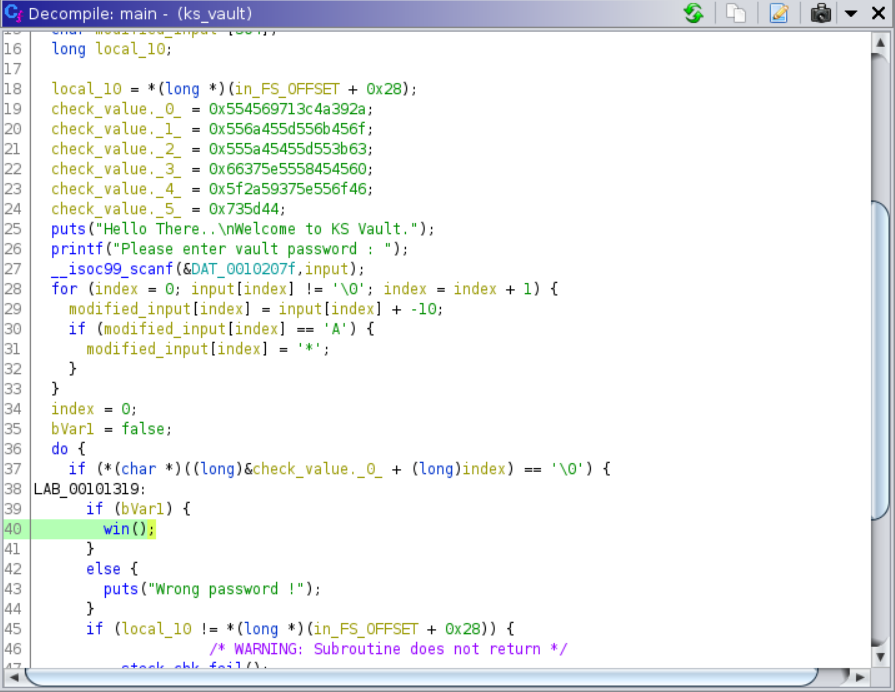If modified value is equal to “A”, it change into “”. Finally it check the modified input with some strings. Let’s make the script, We have value which is compare with modified input. So adding 10 in compare value if the compare value is “”, then add “A” and 10.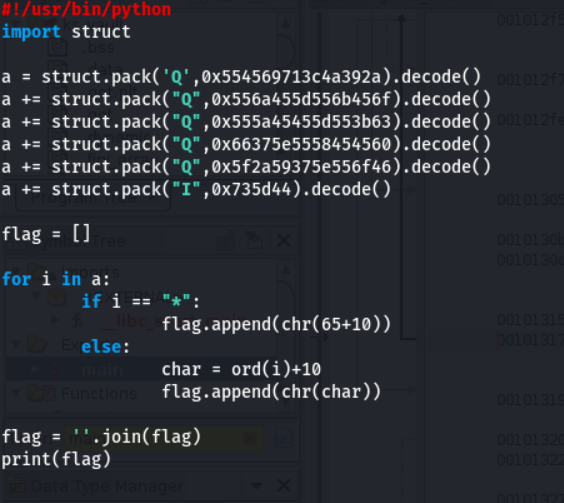Finally it print the flag. Execute the script and check the flag is correct or wrong.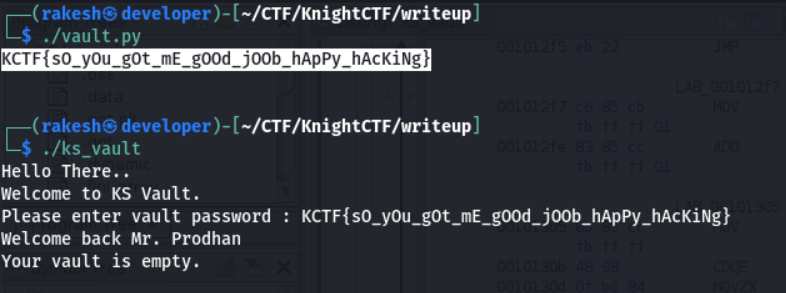The Flag is `KCTF{s0_y0u_g0t_mE_gOOd_jOOb_hApPy_hAcKiNg}`

### Switch Bank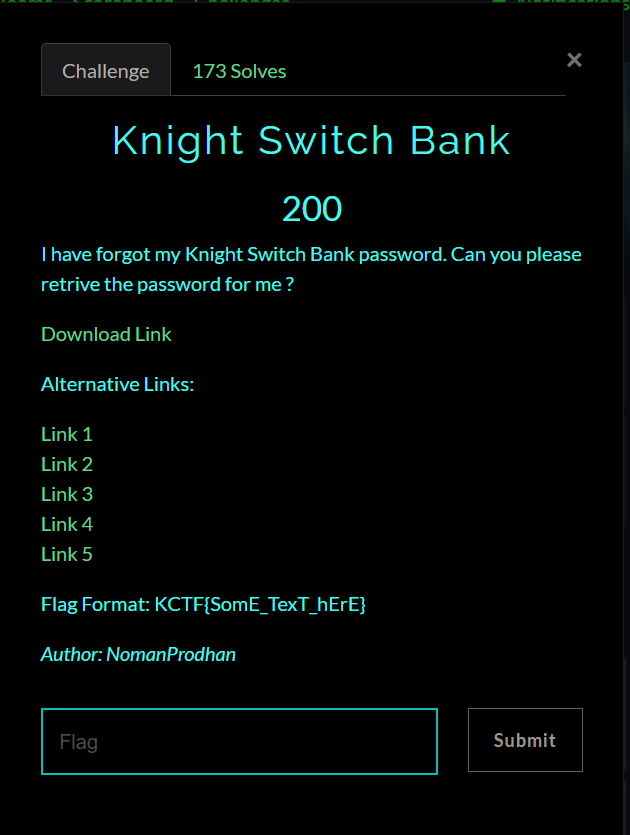##### Basic Info
###### File command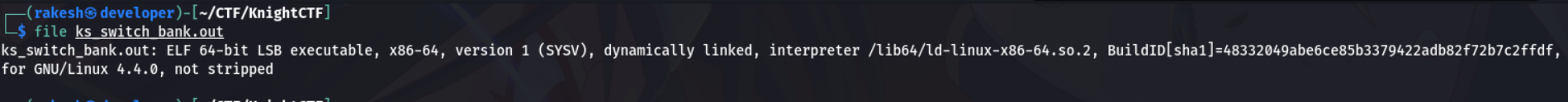It’s an ELF 64bit executable and not stripped binary.

###### Strings command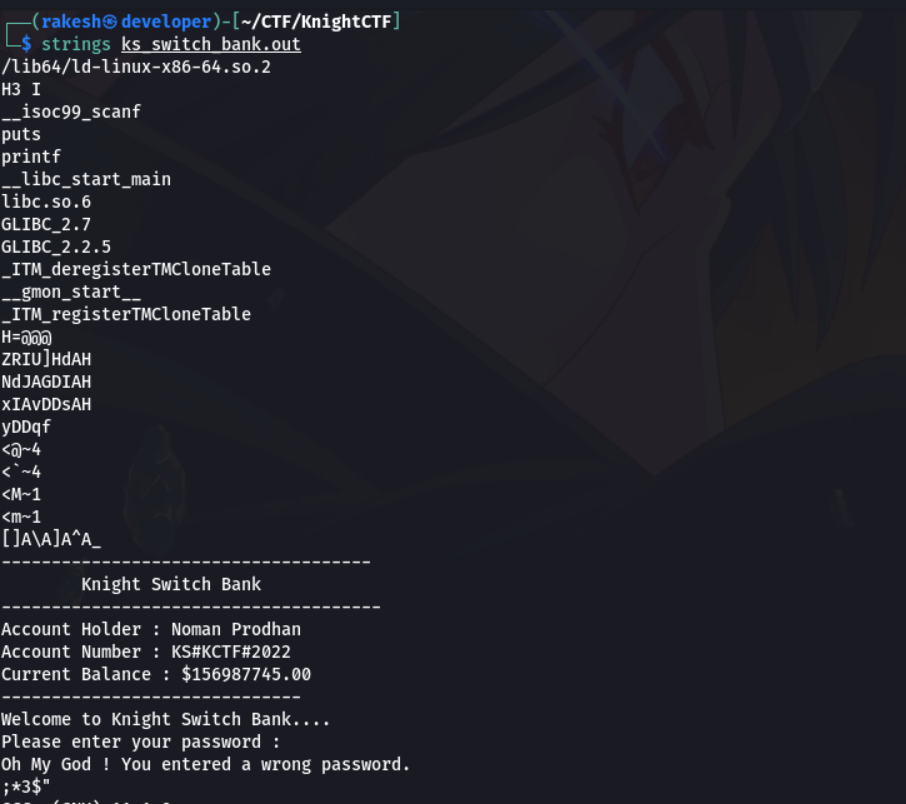Nothing interesting. Now run the binary and see how it works. It print Knight Switch Bank message and ask for password.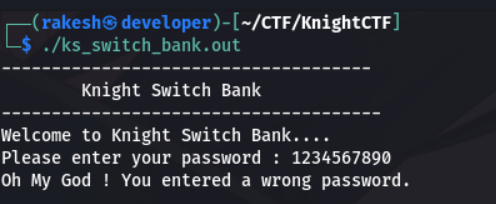Enter 1234567890 as input, it gives wrong password. Load the binary in ghidra to analysis. Look at the main function.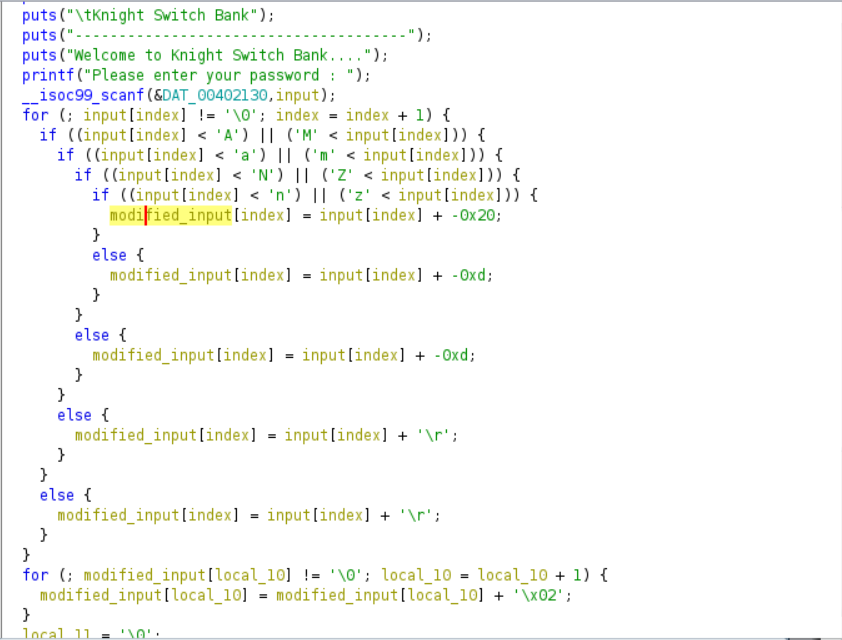It take a input from user. There is one for loop and four if conditions,The for loop iterate every single character into if conditions. The First condition check if input is less than “A” or greater than “M”, if it failed, it subtract 13 from input. Otherwise it goes to second if condition. The Second if condition check the input is less than ‘a’ or greater than ‘m’, if it failed, it subtract 13 from input. If the condition is true, it goes to third if condition. The Third if condition check the input is less than ‘N’ or greater than ‘Z’. If the condition failed, it add 13 in input, if it true it goes to fourth if condition. The Fourth if condition check the input is less than ‘n’ or greater than ‘z’. If the condition failed, it add 13 in input. Otherwise it add 32 in input. Finally it add 2 in modified input and check with some string. Let’s make a script.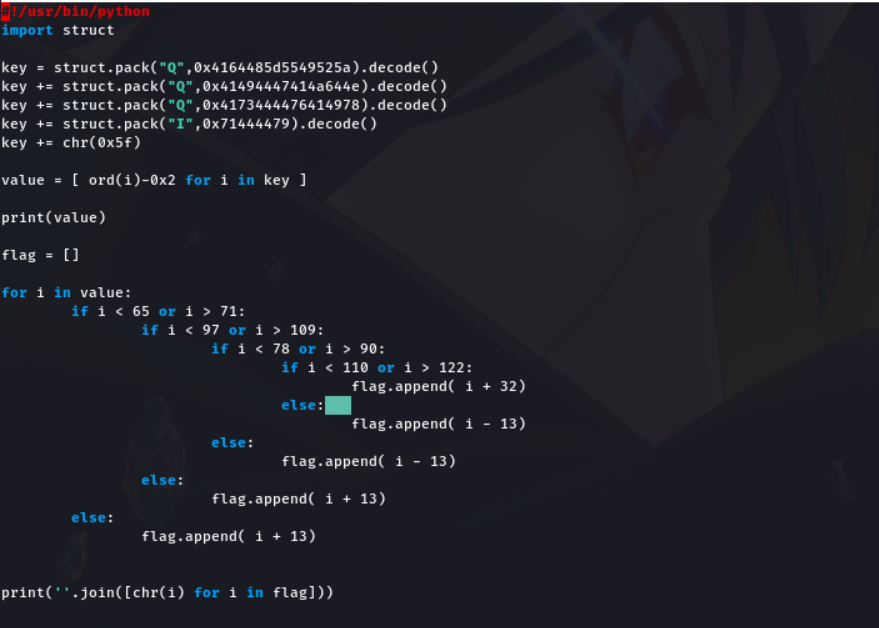We have the compare string, subtract 2 from the compare string. And write if condition as same as in the challenge binary, but change arithmetic operations like if the challenge add 13 in input, we have to subtract 13 from input ( Change the arithmetic signs ). Now run the script and check the output is correct or wrong.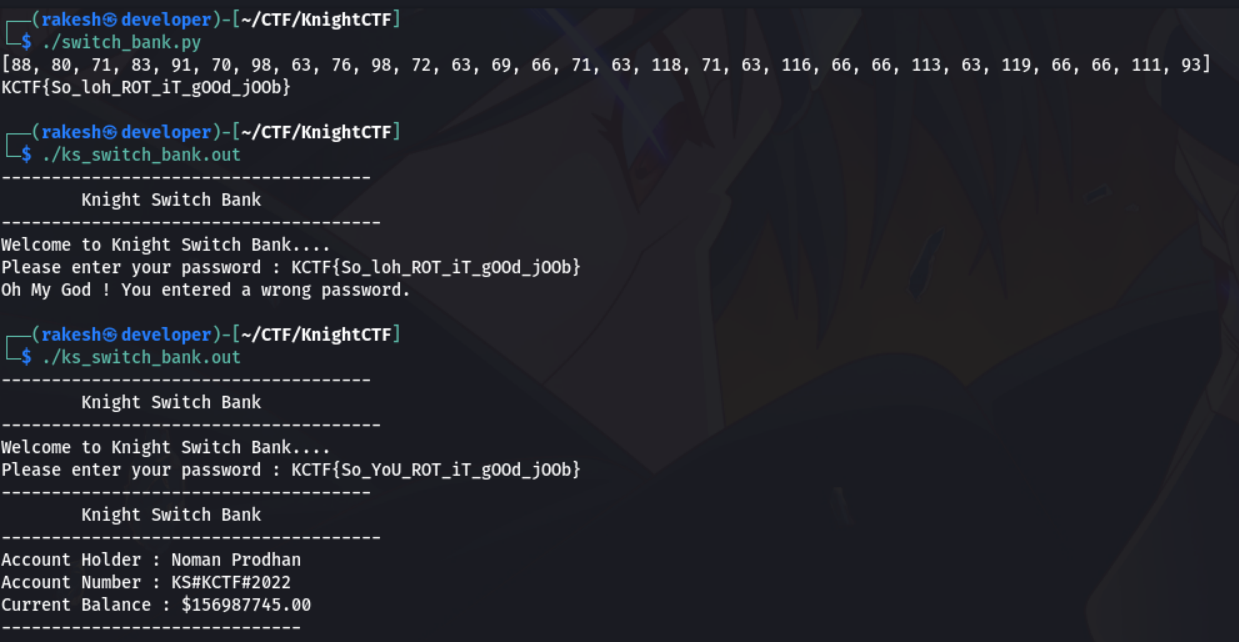The output is KCTF{So_loh_ROT_iT_gOOd_jOOb}. Now run the challenge binary with the script output, but it gives wrong password. By guessing change “loh” into “YoU”, now check it again.

Yeah!! This time the Flag is correct. The flag is `KCTF{So_YoU_ROT_iT_gOOd_jOOb}`